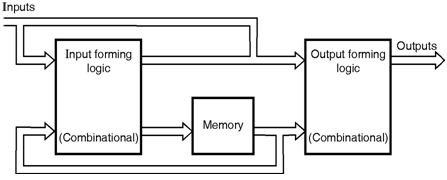Home > finite state machines > State Machine Fundamentals > State Machine

State Machine :

A state machine is a system that can be described in terms of a set of states that the system may enter. The next state reached depends on the inputs and the present state. The outputs also depend on inputs and the present state. A state may be a set of values measured at various points in a circuit. A simple flip-flop has two states in which it can exist. A large majority of practical state machines use locked flip-flops as the storage elements. The code that defines each state then corresponds directly to the code contain by the flip-flops. The general model of the sequential machine is shown in figure below.General model of sequential circuit
This model is also called the Mealy machine after the man who first proposed the model. The input forming logic (IFL) and the output forming logic (OFL) sections are made up of combinational logic circuits. The memory section contains the state of the system. A slight variation of the Mealy machine is the Moore machine which uses only the memory to drive the OFL. In this case, the output is a function of only the state of the system. When a variable is clock-driven, it is considered a synchronous variable. If a machine changes state in response to the clock and all inputs are synchronous, we will classify that circuit as a synchronous system. If the state changes occur in response to the clock, but one or more inputs are not clock-driven, the machine will be called a mainly synchronous system. If the state changes are input-driven rather than clock-driven, the system is an asynchronous one.